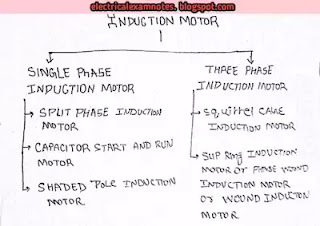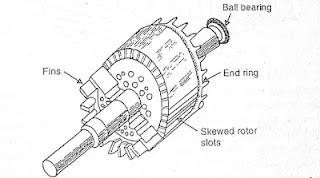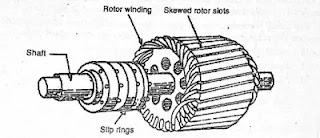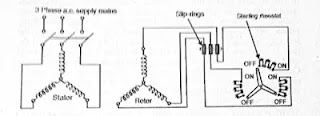# Three phase induction motor,construction,types of three phase induction motor

Hello guys how are you? Hope you are all good and healthy.

So guys we have discussed about the construction, working and types of induction motor in the previous articles.

• Three phase induction motor
• Construction and working of three phase induction motor
• Various types of three phase induction motor.
• Reversal of direction of it.

As guys we have discussed that there are two types of induction motor that is single phase induction motor and three phase induction motors.

To learn about the single phase induction motor click on the link given below: -

But guys I will prefer you to learn first about the construction and working of the induction motor then learn about the single phase induction motor.

So guys we have discussed about :Fig. Types of induction motor

As shown in above diagram I have given you link of both about induction motor construction, working of induction motor and single phase induction motor.

Now we will discuss about the three phase induction motor.

# Three phase induction motor: -

Now a days three phase induction motor is widely used for the industrial purpose due to their low cost, robust and simple in construction and high efficiency.

## WORKING OF THE THREE PHASE INDUCTION MOTOR: -

Let understand this in some steps: -

1. When three phase supply is given to the stator an current flow through the stator winding which produce rotating magnetic field.
2. This rotating magnetic field links with the rotor conductor and according to the electromagnetic induction an emf is induced in the rotor bars or conductors and as we know that the bars are short circuited by the end rings so an current flow through the rotor.
3. This rotor current produces its own magnetic field which opposes the main magnetic field (according to Lenz law).
4. Hence a current carrying conductor (means rotor) is placed in the varying magnetic field (rotating magnetic field) a torque is produced in it (in the rotor). And the rotor starts to rotate in the direction of rotating magnetic field.

So guys we have seen in fig. 1 that,

## There are two types of  three phase induction motor: -

• Squirrel cage induction motor
• Slip ring induction motor or phase wound induction motor or wound induction motor

So let’s come to discuss about types of three phase induction motors.

## Squirrel cage induction motor: -

As the name suggests squirrel cage rotor is used in this type of motor. Squirrel cage is the rotor in which number of slots are provide and copper bars are inserted in these slots at the end they are short circuited by end rings. This type three phase induction motor is widely used. Below fig shows the rotor of the squirrel cage induction motor.Fig. Squirrel cage rotor

In this motor when supply is given, stator flux is produced which is rotating in nature. So this rotating magnetic flux will cut the rotor hence an emf will induce in the rotor. As we also known that the rotor is short circuited (make a close path) a current will also produce in it. So the rotor will starts to rotate.

It is noted here that the rotor always rotates at the speed less than the speed of rotating magnetic field (synchronous speed ).

## Slip ring induction motor :-

As the name suggests in this type of motor three slip rings are used to which rotor windings are connected. The slip ring is a close small circular ring placed on the one side of the shaft of the motor. The brushes on this motor are externally connected to a rheostat (variable resistance). At the starting time, the entire resistance is included in the rotor circuit and the resistance is gradually removed from the circuit until it pick up it’s speed. And when the rotor picks up the speed the whole resistance is removed from the rotor circuit.Fig. Slip ring induction motor rotor

How rotor resistance removed from the rotor circuit?

So we know that the rotor windings are connected to the slip rings and when the rotor pics up, its speed than the rotor winding is short circuited by these slip rings. The slip rings are short circuited by inserting a metal collar along with the shaft.Fig. Slip ring induction motor circuit diagram

Note :- Physically rotor resistance is not removed when we add or inserted a metal collar along with the shaft it connects with the rotor winding or connects all three slip rings to each other and short, these slip rings and we know that the current will flow to that path where low resistance is offered to it. So as compared to external resistance, metal collar resistance is low so it starts flowing through it and short circuited the windings like squirrel cage rotor.

Now let us discuss about the

## SLIP OF INDUCTION MOTOR :-

As we have studied above that the rotor speed of an induction motor is not more than the speed of the rotating magnetic field. The rotor speed will be always less than the stator speed. So the difference between the speed of the rotating magnetic field of the induction motor and the rotor speed, expressed as the fraction of rotating magnetic field ( synchronous speed ) is known as slip. The slip is given by,

Slip ( s ) = Ns–N/ Ns

Where,

Ns = synchronous speed
N= rotor speed

It is noted here that the synchronous motor should always run speed less than the speed of synchronous speed.

So,
Why induction motor should run at synchronous speed?

So let us understand this..

When the supply given to the stator, the rotating magnetic field is produced which produce an emf in the rotor due to which a current flow in the rotor. Due to this current it produces a magnetic field which follows the rotating magnetic field (stator field). Let assume if the rotor rotates at the speed of synchronous speed (means rotor magnetic field speed is equal to the speed of the rotating magnetic field) than the rotating magnetic field will not cut the rotor (in this condition rotating magnetic field will be in parallel to rotor magnetic field). Due to which any emf will not induce in the rotor and also no current will flow. No current means no rotor magnetic field which further means that there is no any magnetic field which follow the rotating magnetic field so rotor will not rotate.

So that’s why the rotor should not rotate at the synchronous speed.

So now let's come to learn about the

## Significance (importance) of slip: -

Let me tell you here that the slip or the difference between the rotor speed and the synchronous speed  of the flux is directly proportional to the induced emf. And also torque is directly proportional to the slip. Therefore more the slip more will be the torque.

At the no load the induction motors require low torque to rotate so the slip will be low and if the load is put on the motor, the torque requires more to rotate so slip will be more to get the more torque.

Therefore, we can say that the slip adjust the torque requirement of the induction motor.

So To adjust the motor according to its torque requirements slip is necessary in the induction motor.

And slip is also required to produce emf in the rotor if there is no slip there is no emf and current in the rotor of the induction motor.

# Reversal of direction of rotation of induction motor: -

The direction of rotation of three phase induction motors can be reversed by reversing the direction of rotation of rotating magnetic field. Which can be done by interchanging the any two phases of the supply. Means if R, Y, B is the phases than interchange R and B to each other.

If you like the article then share with your friends.

If you have any doubt then tell me in a comment.

Thank you

Hope article helps you.